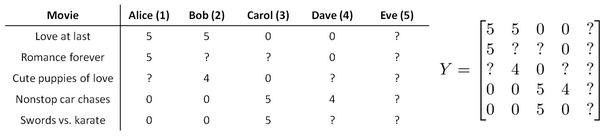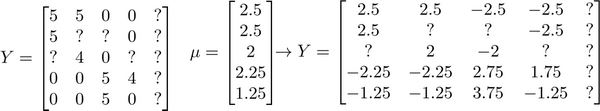# 第9周

## 十五、异常检测(Anomaly Detection)

### 15.1 问题的动机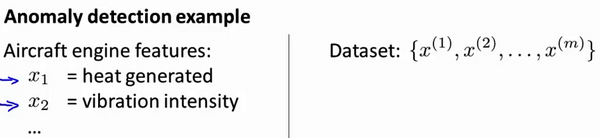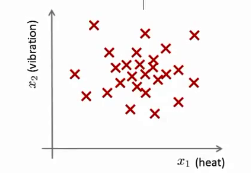$x^{(i)} = {用户的第i个活动特征}$

### 15.2 高斯分布

$\sigma^2=\frac{1}{m}\sum\limits_{i=1}^{m}(x^{(i)}-\mu)^2$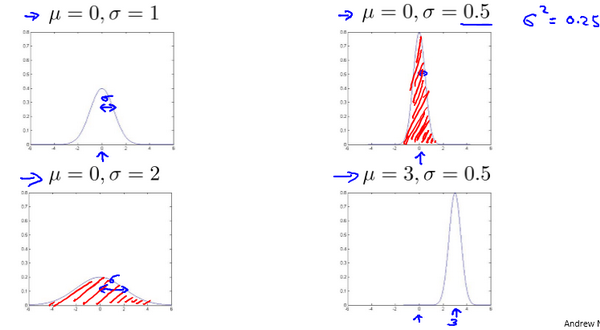### 15.3 算法

$\mu_j=\frac{1}{m}\sum\limits_{i=1}^{m}x_j^{(i)}$

$\sigma_j^2=\frac{1}{m}\sum\limits_{i=1}^m(x_j^{(i)}-\mu_j)^2$

$p(x)=\prod\limits_{j=1}^np(x_j;\mu_j,\sigma_j^2)=\prod\limits_{j=1}^1\frac{1}{\sqrt{2\pi}\sigma_j}exp(-\frac{(x_j-\mu_j)^2}{2\sigma_j^2})$

$p(x) < \varepsilon$时，为异常。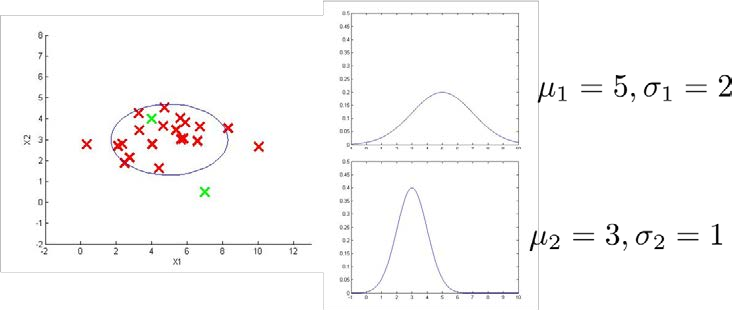### 15.4 开发和评价一个异常检测系统

6000台正常引擎的数据作为训练集

2000台正常引擎和10台异常引擎的数据作为交叉检验集

2000台正常引擎和10台异常引擎的数据作为测试集

1. 根据测试集数据，我们估计特征的平均值和方差并构建$p(x)$函数
2. 对交叉检验集，我们尝试使用不同的$\varepsilon$值作为阀值，并预测数据是否异常，根据F1值或者查准率与查全率的比例来选择 $\varepsilon$
3. 选出 $\varepsilon$ 后，针对测试集进行预测，计算异常检验系统的$F1$值，或者查准率与查全率之比

### 15.6 选择特征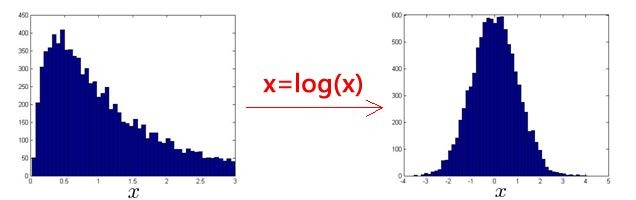### 15.7 多元高斯分布（选修）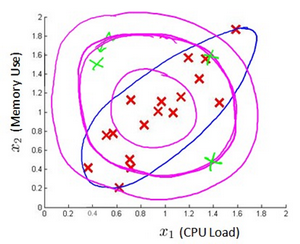$\mu=\frac{1}{m}\sum_{i=1}^mx^{(i)}$

$\Sigma = \frac{1}{m}\sum_{i=1}^m(x^{(i)}-\mu)(x^{(i)}-\mu)^T=\frac{1}{m}(X-\mu)^T(X-\mu)$

$|\Sigma|$是定矩阵，在 Octave 中用 det(sigma)计算

$\Sigma1$ 是逆矩阵，下面我们来看看协方差矩阵是如何影响模型的：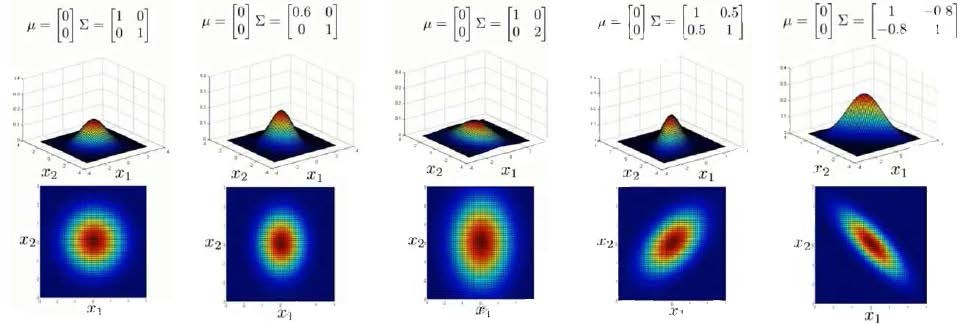1. 是一个一般的高斯分布模型
2. 通过协方差矩阵，令特征1拥有较小的偏差，同时保持特征2的偏差
3. 通过协方差矩阵，令特征2拥有较大的偏差，同时保持特征1的偏差
4. 通过协方差矩阵，在不改变两个特征的原有偏差的基础上，增加两者之间的正相关性
5. 通过协方差矩阵，在不改变两个特征的原有偏差的基础上，增加两者之间的负相关性

### 15.8 使用多元高斯分布进行异常检测（可选）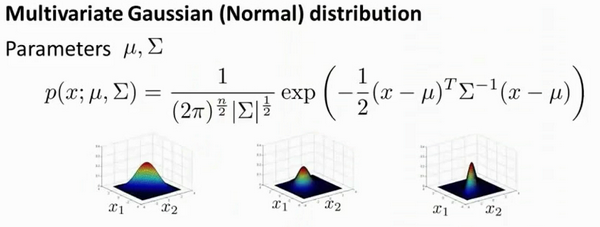$\mu=\frac{1}{m}\sum_{i=1}^{m}x^{(i)}$ 并设置$\Sigma$$\Sigma=\frac{1}{m}\sum_{i=1}^{m}(x^{(i)}-\mu)(x^{(i)}-\mu)^T$ 这其实只是当我们使用PCA算法时候，有 $\Sigma$ 时写出来。所以你只需插入上述两个公式，这会给你你估计的参数 $\mu$ 和你估计的参数 $\Sigma$。所以，这里给出的数据集是你如何估计 $\mu$$\Sigma$。让我们以这种方法而只需将其插入到异常检测算法。那么，我们如何把所有这一切共同开发一个异常检测算法？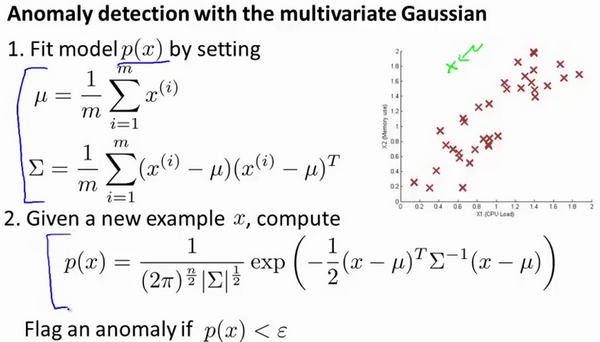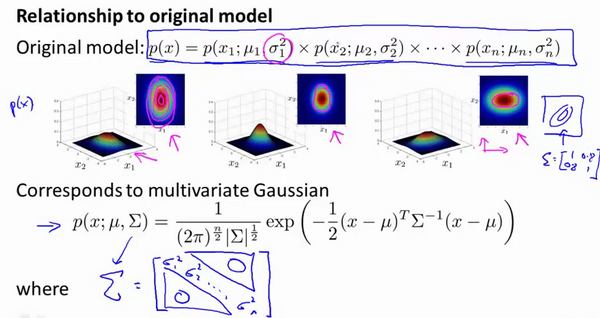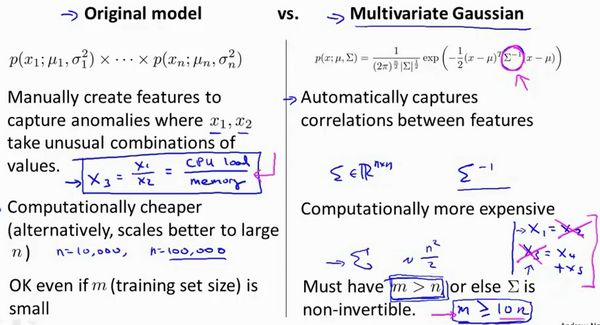## 十六、推荐系统(Recommender Systems)

### 16.1 问题形式化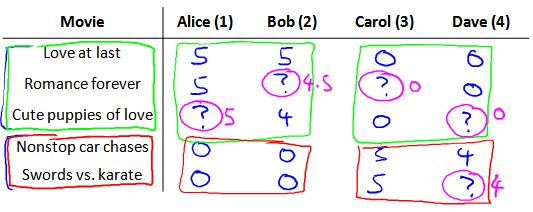$n_u$ 代表用户的数量

$n_m$ 代表电影的数量

$r(i, j)$ 如果用户j给电影 $i$ 评过分则 $r(i,j)=1$

$y^{(i, j)}$ 代表用户 $j$ 给电影i的评分

$m_j$代表用户 $j$ 评过分的电影的总数

### 16.2 基于内容的推荐系统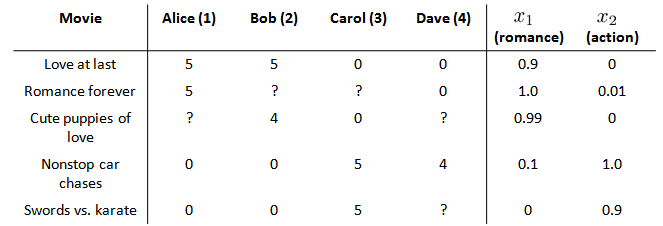$\theta^{(j)}$用户 $j$ 的参数向量

$x^{(i)}$电影 $i$ 的特征向量

### 16.3 协同过滤

1. 初始 $x^{(1)},x^{(1)},...x^{(nm)},\ \theta^{(1)},\theta^{(2)},...,\theta^{(n_u)}$为一些随机小值
2. 使用梯度下降算法最小化代价函数
3. 在训练完算法后，我们预测$(\theta^{(j)})^Tx^{(i)}$为用户 $j$ 给电影 $i$ 的评分

### 16.5 向量化：低秩矩阵分解

1. 当给出一件产品时，你能否找到与之相关的其它产品。
2. 一位用户最近看上一件产品，有没有其它相关的产品，你可以推荐给他。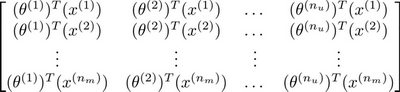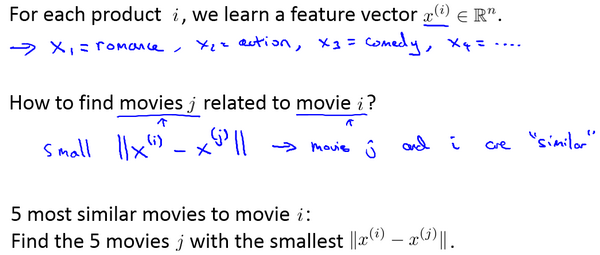### 16.6 推行工作上的细节：均值归一化